Скачать презентацию Primal-dual Approximation Algorithms for Integral Flow and Multicut

059736b25123f8199ab5207aec3b9f03.ppt

• Количество слайдов: 28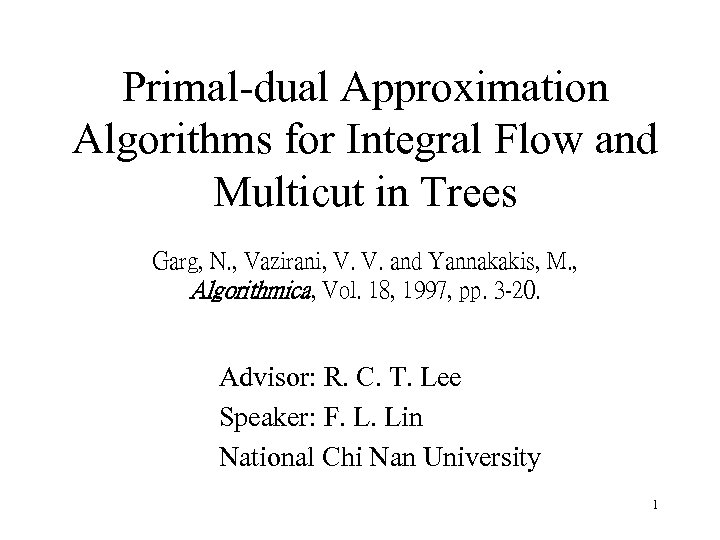Primal-dual Approximation Algorithms for Integral Flow and Multicut in Trees Garg, N. , Vazirani, V. V. and Yannakakis, M. , Algorithmica, Vol. 18, 1997, pp. 3 -20. Advisor: R. C. T. Lee Speaker: F. L. Lin National Chi Nan University 1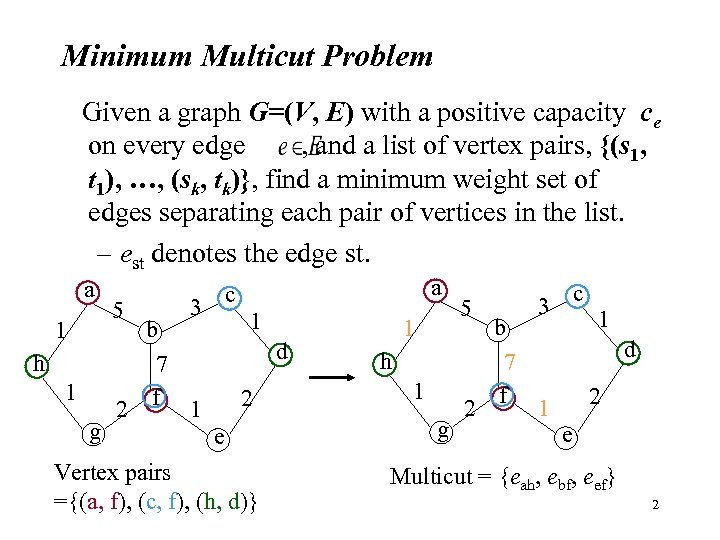Minimum Multicut Problem Given a graph G=(V, E) with a positive capacity ce on every edge , and a list of vertex pairs, {(s 1, t 1), …, (sk, tk)}, find a minimum weight set of edges separating each pair of vertices in the list. – est denotes the edge st. a 1 5 h 1 g 2 b 7 f c 3 a 1 d 2 1 e Vertex pairs ={(a, f), (c, f), (h, d)} 1 5 h 1 g 2 b 7 f c 3 1 d 2 1 e Multicut = {eah, ebf, eef} 2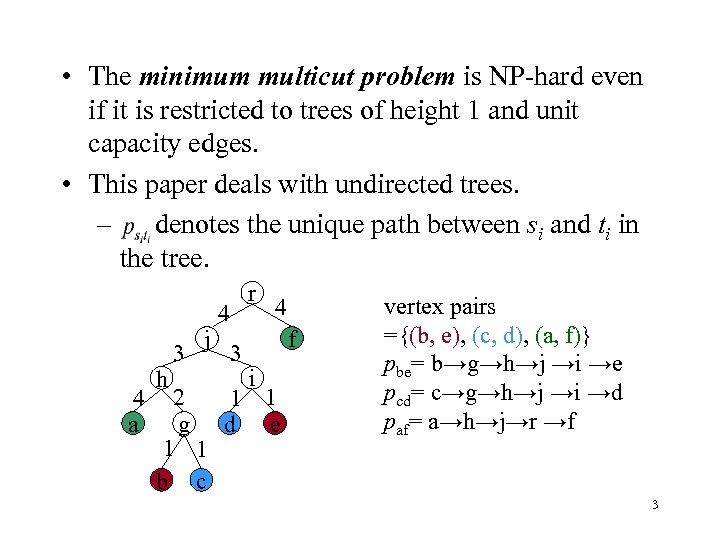• The minimum multicut problem is NP-hard even if it is restricted to trees of height 1 and unit capacity edges. • This paper deals with undirected trees. – denotes the unique path between si and ti in the tree. 4 a h 3 j 4 3 r 4 f i 2 1 1 g d e 1 1 b c vertex pairs ={(b, e), (c, d), (a, f)} pbe= b→g→h→j →i →e pcd= c→g→h→j →i →d paf= a→h→j→r →f 3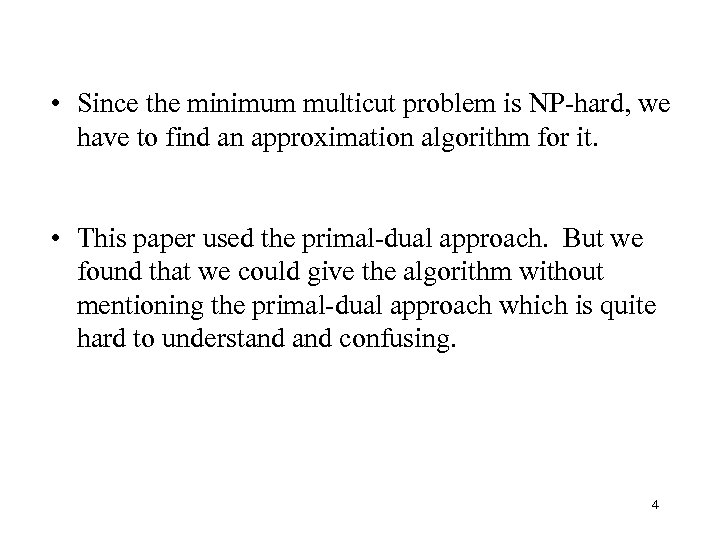• Since the minimum multicut problem is NP-hard, we have to find an approximation algorithm for it. • This paper used the primal-dual approach. But we found that we could give the algorithm without mentioning the primal-dual approach which is quite hard to understand confusing. 4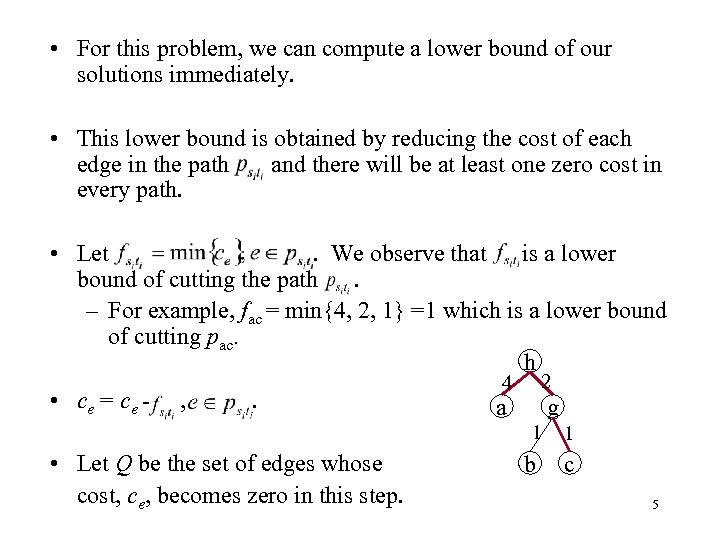• For this problem, we can compute a lower bound of our solutions immediately. • This lower bound is obtained by reducing the cost of each edge in the path and there will be at least one zero cost in every path. • Let. We observe that is a lower bound of cutting the path. – For example, fac = min{4, 2, 1} =1 which is a lower bound of cutting pac. h • ce = ce - , . 2 4 a g 1 • Let Q be the set of edges whose cost, ce, becomes zero in this step. 1 b c 5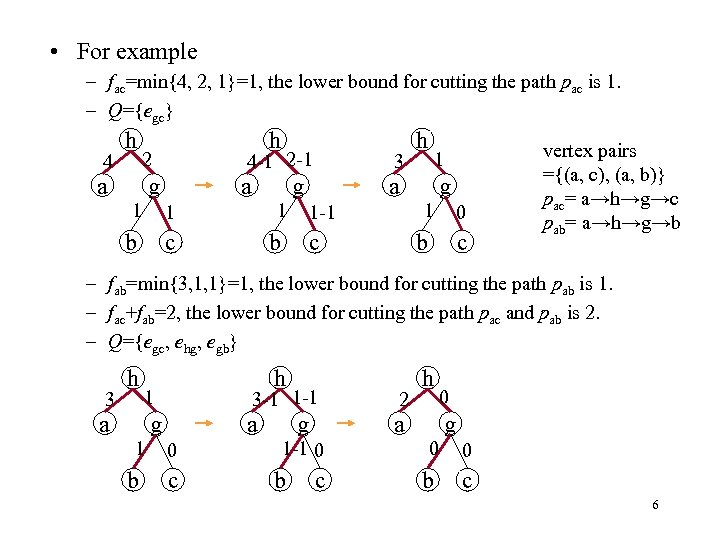• For example – fac=min{4, 2, 1}=1, the lower bound for cutting the path pac is 1. – Q={egc} 4 h h 2 a 4 -1 2 -1 g a 3 1 1 b c b 1 a g 1 h g 1 c 0 b 1 -1 c vertex pairs ={(a, c), (a, b)} pac= a→h→g→c pab= a→h→g→b – fab=min{3, 1, 1}=1, the lower bound for cutting the path pab is 1. – fac+fab=2, the lower bound for cutting the path pac and pab is 2. – Q={egc, ehg, egb} 3 h h 1 a 3 -1 1 -1 g a 1 0 b c g 1 -1 0 b c 2 h 0 a g 0 0 b c 6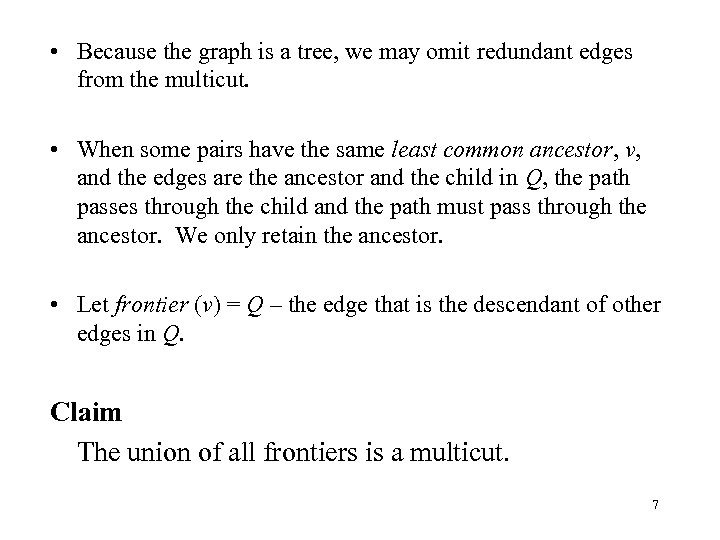• Because the graph is a tree, we may omit redundant edges from the multicut. • When some pairs have the same least common ancestor, v, and the edges are the ancestor and the child in Q, the path passes through the child and the path must pass through the ancestor. We only retain the ancestor. • Let frontier (v) = Q – the edge that is the descendant of other edges in Q. Claim The union of all frontiers is a multicut. 7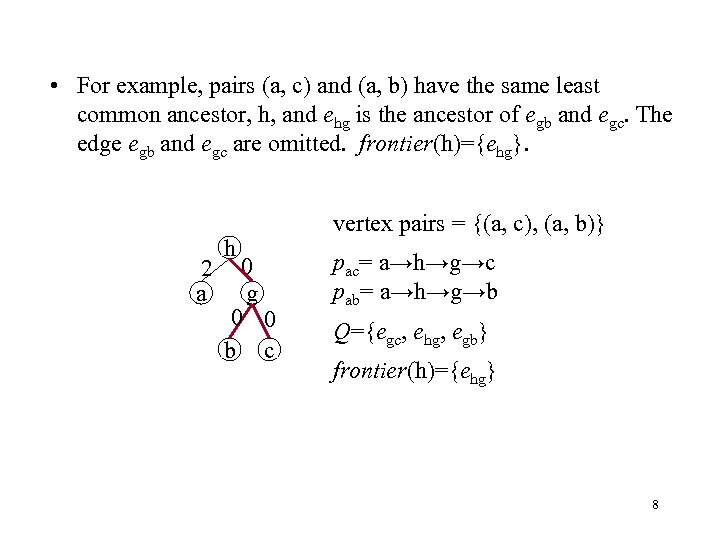• For example, pairs (a, c) and (a, b) have the same least common ancestor, h, and ehg is the ancestor of egb and egc. The edge egb and egc are omitted. frontier(h)={ehg}. 2 a h 0 g 0 0 b c vertex pairs = {(a, c), (a, b)} pac= a→h→g→c pab= a→h→g→b Q={egc, ehg, egb} frontier(h)={ehg} 8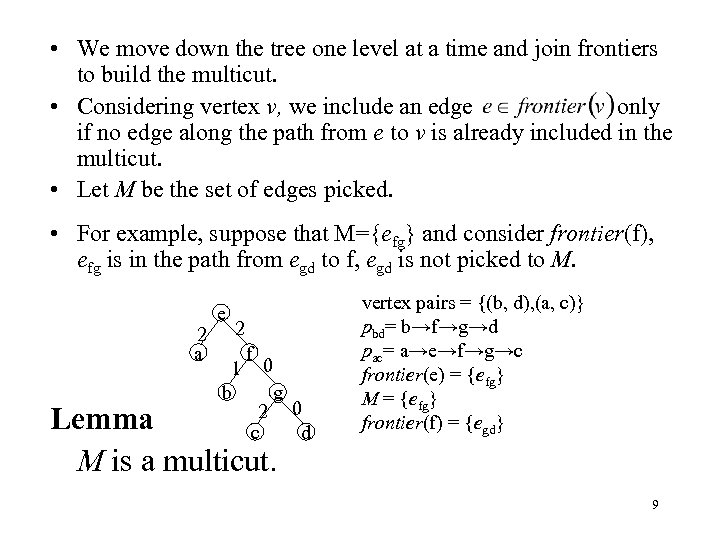• We move down the tree one level at a time and join frontiers to build the multicut. • Considering vertex v, we include an edge only if no edge along the path from e to v is already included in the multicut. • Let M be the set of edges picked. • For example, suppose that M={efg} and consider frontier(f), efg is in the path from egd to f, egd is not picked to M. 2 a e 2 1 b f 0 g 2 0 c d Lemma M is a multicut. vertex pairs = {(b, d), (a, c)} pbd= b→f→g→d pac= a→e→f→g→c frontier(e) = {efg} M = {efg} frontier(f) = {egd} 9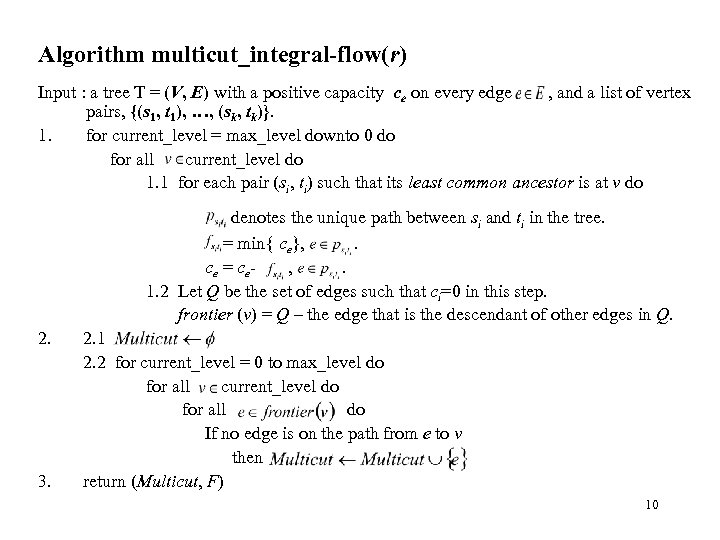Algorithm multicut_integral-flow(r) Input : a tree T = (V, E) with a positive capacity ce on every edge , and a list of vertex pairs, {(s 1, t 1), …, (sk, tk)}. 1. for current_level = max_level downto 0 do for all current_level do 1. 1 for each pair (si, ti) such that its least common ancestor is at v do denotes the unique path between si and ti in the tree. = min{ ce}, . ce = ce, . 1. 2 Let Q be the set of edges such that ci=0 in this step. frontier (v) = Q – the edge that is the descendant of other edges in Q. 2. 3. 2. 1 2. 2 for current_level = 0 to max_level do for all current_level do for all do If no edge is on the path from e to v then return (Multicut, F) 10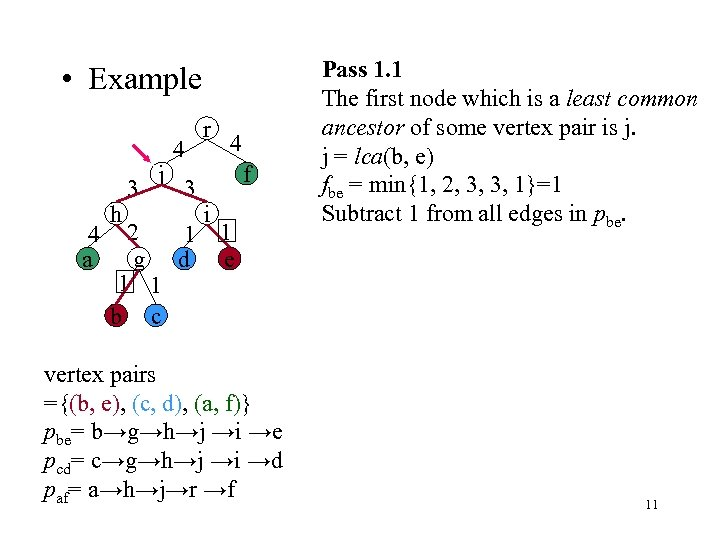• Example 4 a h 3 j 4 3 r 4 f i 2 1 1 g d e 1 1 b c vertex pairs ={(b, e), (c, d), (a, f)} pbe= b→g→h→j →i →e pcd= c→g→h→j →i →d paf= a→h→j→r →f Pass 1. 1 The first node which is a least common ancestor of some vertex pair is j. j = lca(b, e) fbe = min{1, 2, 3, 3, 1}=1 Subtract 1 from all edges in pbe. 11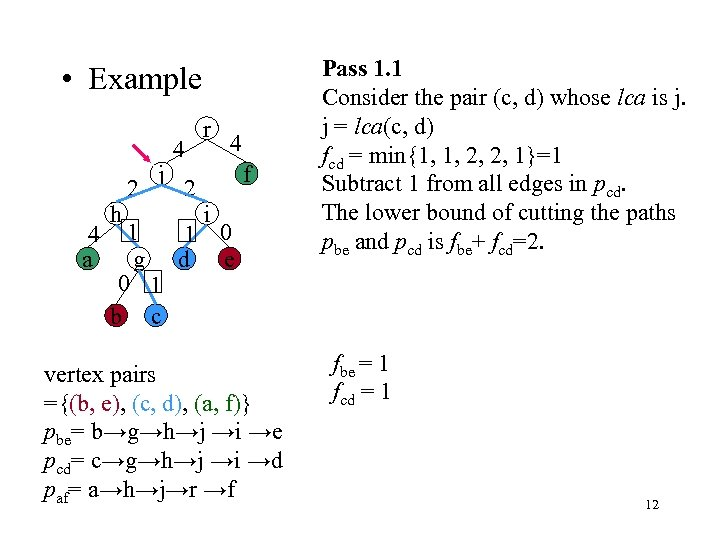• Example 4 a h 2 j 4 2 r 4 f i 1 1 0 g d e 0 1 b c vertex pairs ={(b, e), (c, d), (a, f)} pbe= b→g→h→j →i →e pcd= c→g→h→j →i →d paf= a→h→j→r →f Pass 1. 1 Consider the pair (c, d) whose lca is j. j = lca(c, d) fcd = min{1, 1, 2, 2, 1}=1 Subtract 1 from all edges in pcd. The lower bound of cutting the paths pbe and pcd is fbe+ fcd=2. fbe = 1 fcd = 1 12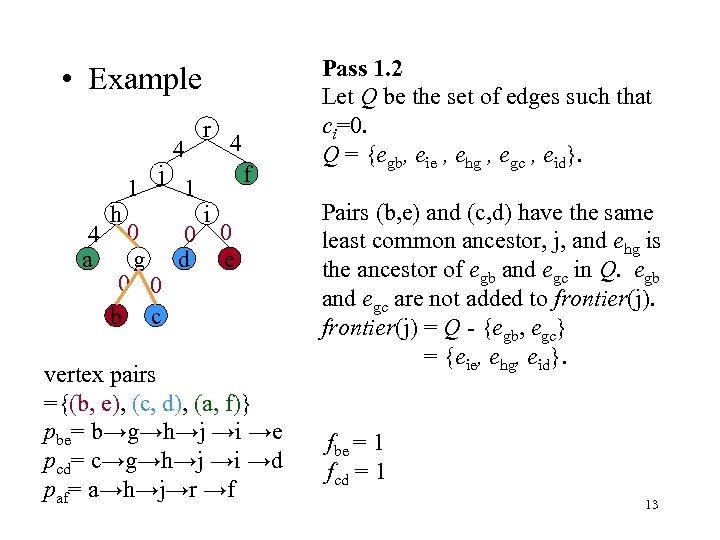• Example 4 a h 1 j 4 1 r 4 f i 0 0 0 g d e 0 0 b c vertex pairs ={(b, e), (c, d), (a, f)} pbe= b→g→h→j →i →e pcd= c→g→h→j →i →d paf= a→h→j→r →f Pass 1. 2 Let Q be the set of edges such that ci=0. Q = {egb, eie , ehg , egc , eid}. Pairs (b, e) and (c, d) have the same least common ancestor, j, and ehg is the ancestor of egb and egc in Q. egb and egc are not added to frontier(j) = Q - {egb, egc} = {eie, ehg, eid}. fbe = 1 fcd = 1 13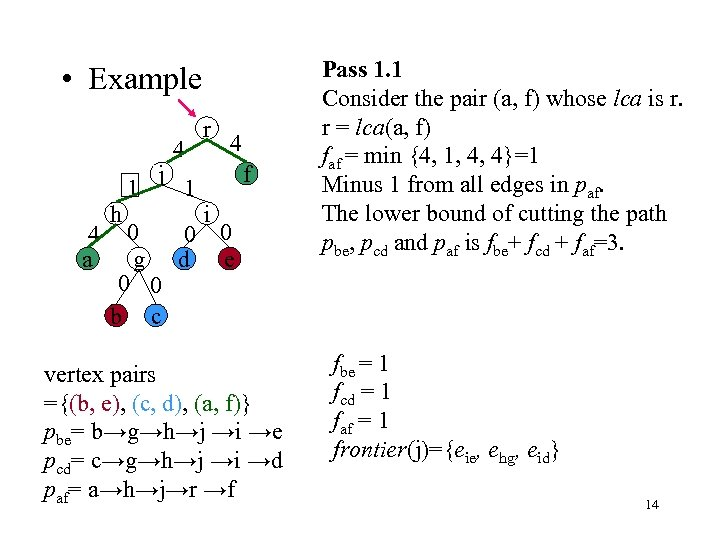• Example 4 a h 1 j 4 1 r 4 f i 0 0 0 g d e 0 0 b c vertex pairs ={(b, e), (c, d), (a, f)} pbe= b→g→h→j →i →e pcd= c→g→h→j →i →d paf= a→h→j→r →f Pass 1. 1 Consider the pair (a, f) whose lca is r. r = lca(a, f) faf = min {4, 1, 4, 4}=1 Minus 1 from all edges in paf. The lower bound of cutting the path pbe, pcd and paf is fbe+ fcd + faf=3. fbe = 1 fcd = 1 faf = 1 frontier(j)={eie, ehg, eid} 14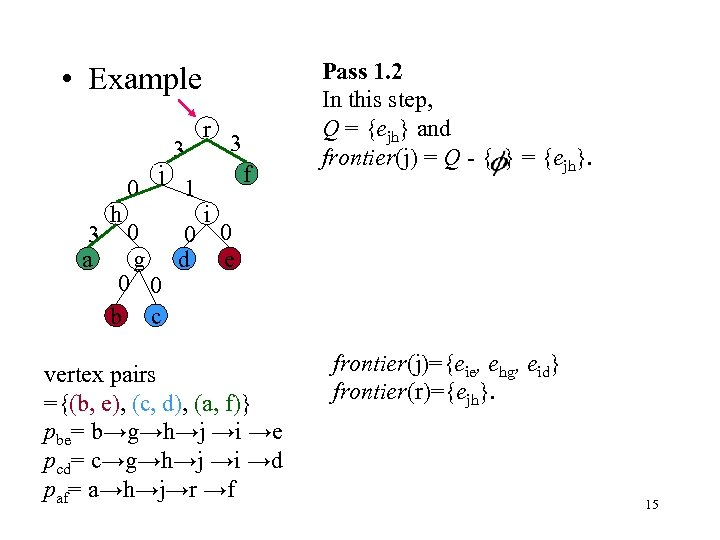• Example 3 a h 0 j 3 1 r 3 f Pass 1. 2 In this step, Q = {ejh} and frontier(j) = Q - { } = {ejh}. i 0 0 0 g d e 0 0 b c vertex pairs ={(b, e), (c, d), (a, f)} pbe= b→g→h→j →i →e pcd= c→g→h→j →i →d paf= a→h→j→r →f frontier(j)={eie, ehg, eid} frontier(r)={ejh}. 15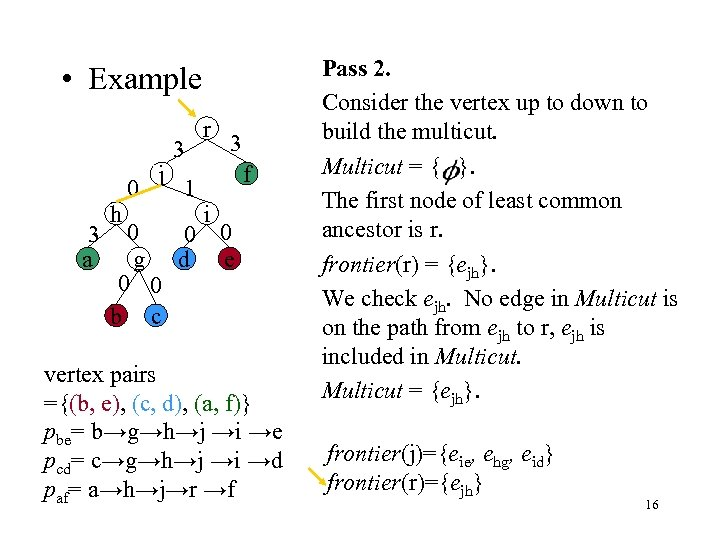• Example 3 a h 0 j 3 1 r 3 f i 0 0 0 g d e 0 0 b c vertex pairs ={(b, e), (c, d), (a, f)} pbe= b→g→h→j →i →e pcd= c→g→h→j →i →d paf= a→h→j→r →f Pass 2. Consider the vertex up to down to build the multicut. Multicut = { }. The first node of least common ancestor is r. frontier(r) = {ejh}. We check ejh. No edge in Multicut is on the path from ejh to r, ejh is included in Multicut = {ejh}. frontier(j)={eie, ehg, eid} frontier(r)={ejh} 16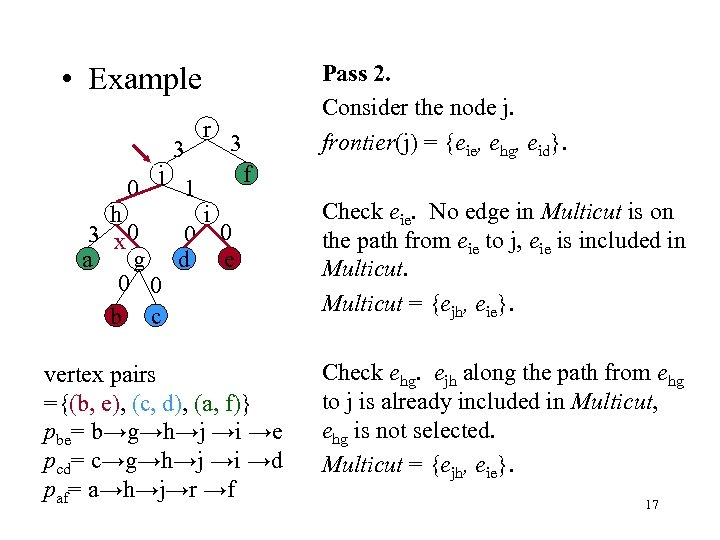Pass 2. Consider the node j. frontier(j) = {eie, ehg, eid}. • Example 0 j 3 1 r 3 f i h 3 x 0 0 0 a g d e 0 0 b c Check eie. No edge in Multicut is on the path from eie to j, eie is included in Multicut = {ejh, eie}. vertex pairs ={(b, e), (c, d), (a, f)} pbe= b→g→h→j →i →e pcd= c→g→h→j →i →d paf= a→h→j→r →f Check ehg. ejh along the path from ehg to j is already included in Multicut, ehg is not selected. Multicut = {ejh, eie}. 17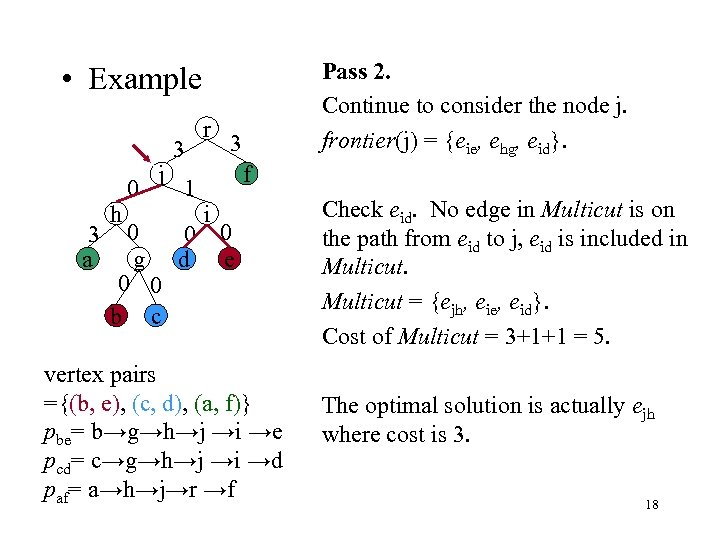Pass 2. Continue to consider the node j. frontier(j) = {eie, ehg, eid}. • Example 3 a h 0 j 3 1 r 3 f i 0 0 0 g d e 0 0 b c vertex pairs ={(b, e), (c, d), (a, f)} pbe= b→g→h→j →i →e pcd= c→g→h→j →i →d paf= a→h→j→r →f Check eid. No edge in Multicut is on the path from eid to j, eid is included in Multicut = {ejh, eie, eid}. Cost of Multicut = 3+1+1 = 5. The optimal solution is actually ejh where cost is 3. 18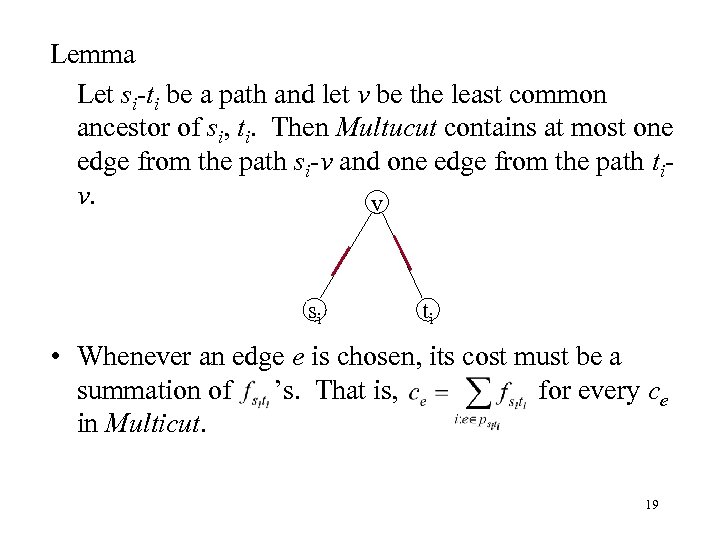Lemma Let si-ti be a path and let v be the least common ancestor of si, ti. Then Multucut contains at most one edge from the path si-v and one edge from the path tiv. v si ti • Whenever an edge e is chosen, its cost must be a summation of ’s. That is, for every ce in Multicut. 19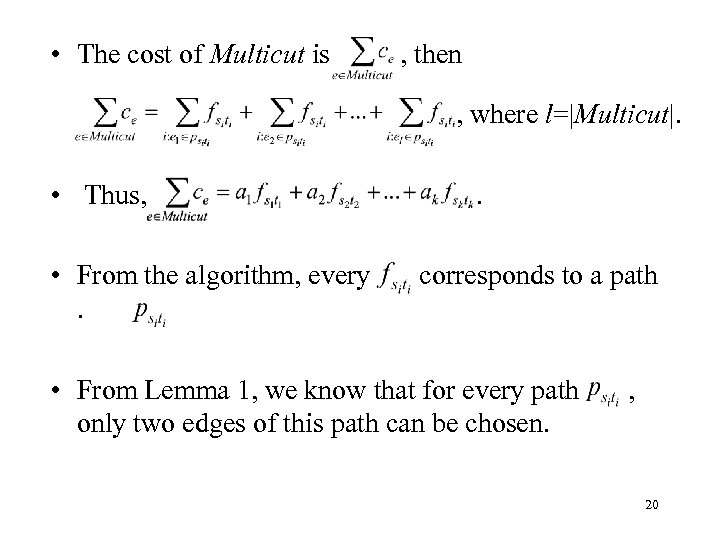• The cost of Multicut is , then , where l=|Multicut|. • Thus, • From the algorithm, every. . corresponds to a path • From Lemma 1, we know that for every path only two edges of this path can be chosen. , 20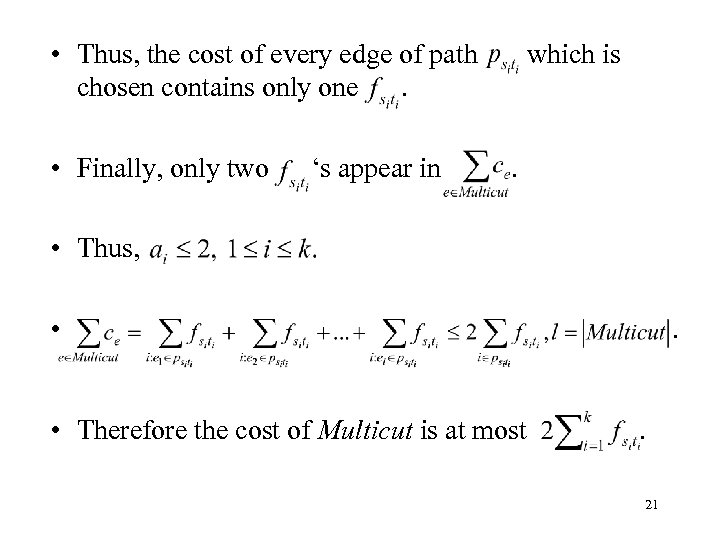• Thus, the cost of every edge of path chosen contains only one. • Finally, only two ‘s appear in which is . • Thus, • • Therefore the cost of Multicut is at most . . 21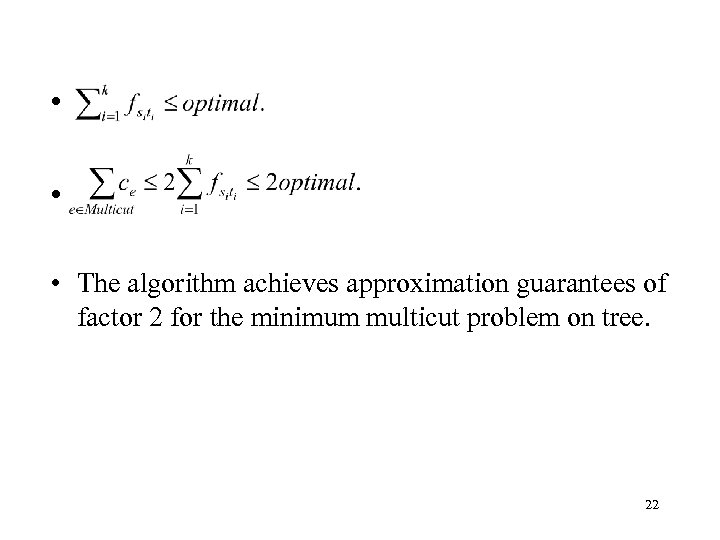• • • The algorithm achieves approximation guarantees of factor 2 for the minimum multicut problem on tree. 22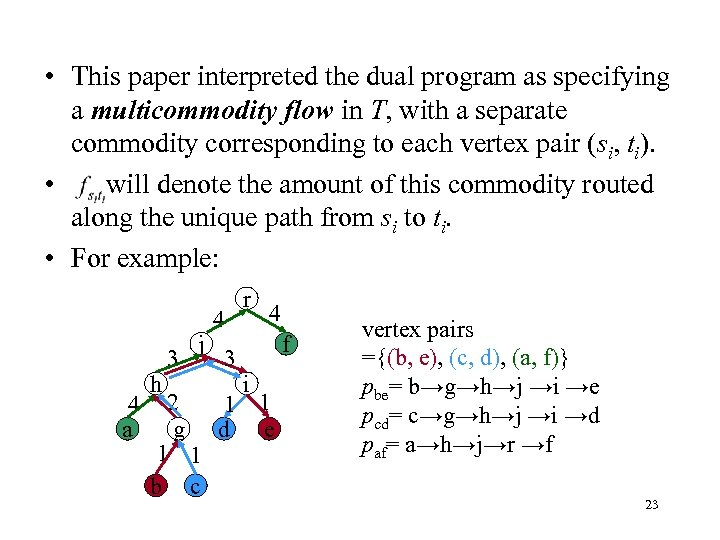• This paper interpreted the dual program as specifying a multicommodity flow in T, with a separate commodity corresponding to each vertex pair (si, ti). • will denote the amount of this commodity routed along the unique path from si to ti. • For example: 4 a h 3 j 4 3 r 4 f i 2 1 1 g d e 1 1 b c vertex pairs ={(b, e), (c, d), (a, f)} pbe= b→g→h→j →i →e pcd= c→g→h→j →i →d paf= a→h→j→r →f 23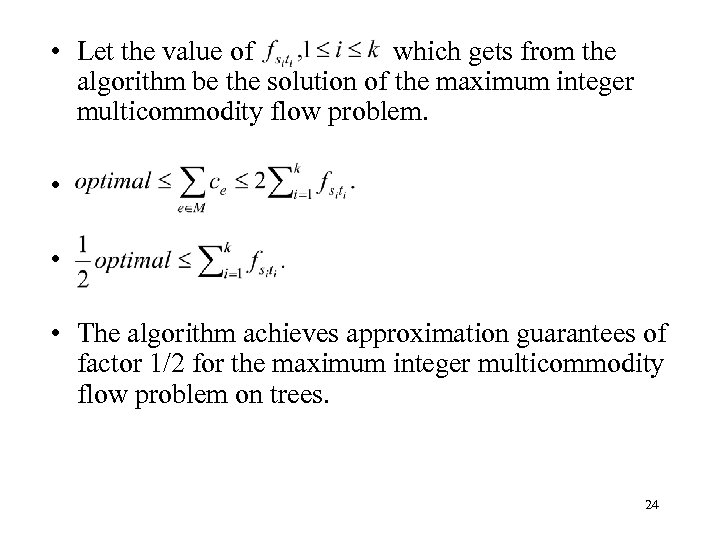• Let the value of which gets from the algorithm be the solution of the maximum integer multicommodity flow problem. • • • The algorithm achieves approximation guarantees of factor 1/2 for the maximum integer multicommodity flow problem on trees. 24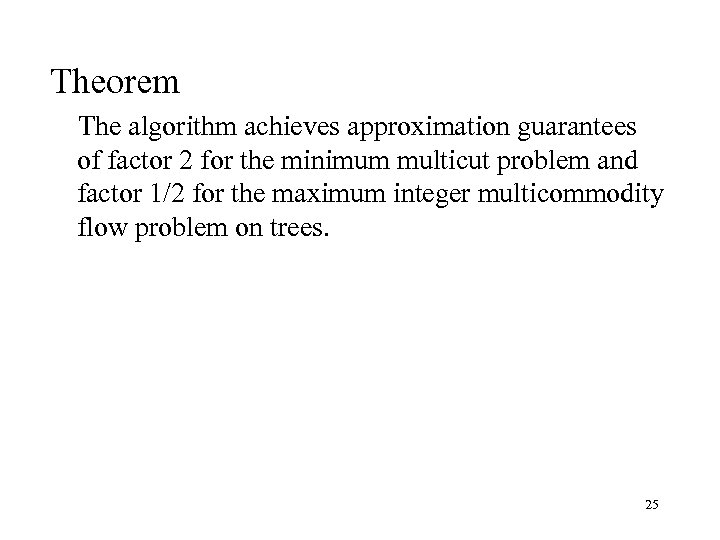Theorem The algorithm achieves approximation guarantees of factor 2 for the minimum multicut problem and factor 1/2 for the maximum integer multicommodity flow problem on trees. 25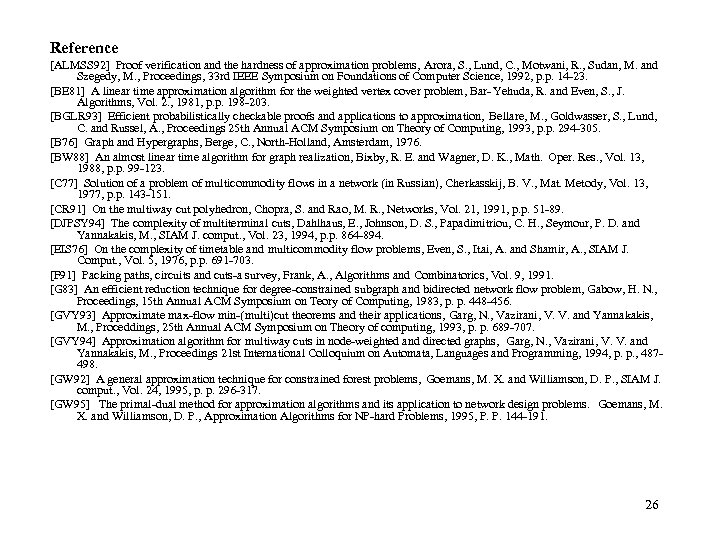Reference [ALMSS 92] Proof verification and the hardness of approximation problems, Arora, S. , Lund, C. , Motwani, R. , Sudan, M. and Szegedy, M. , Proceedings, 33 rd IEEE Symposium on Foundations of Computer Science, 1992, p. p. 14 -23. [BE 81] A linear time approximation algorithm for the weighted vertex cover problem, Bar- Yehuda, R. and Even, S. , J. Algorithms, Vol. 2. , 1981, p. p. 198 -203. [BGLR 93] Efficient probabilistically checkable proofs and applications to approximation, Bellare, M. , Goldwasser, S. , Lund, C. and Russel, A. , Proceedings 25 th Annual ACM Symposium on Theory of Computing, 1993, p. p. 294 -305. [B 76] Graph and Hypergraphs, Berge, C. , North-Holland, Amsterdam, 1976. [BW 88] An almost linear time algorithm for graph realization, Bixby, R. E. and Wagner, D. K. , Math. Oper. Res. , Vol. 13, 1988, p. p. 99 -123. [C 77] Solution of a problem of multicommodity flows in a network (in Russian), Cherkasskij, B. V. , Mat. Metody, Vol. 13, 1977, p. p. 143 -151. [CR 91] On the multiway cut polyhedron, Chopra, S. and Rao, M. R. , Networks, Vol. 21, 1991, p. p. 51 -89. [DJPSY 94] The complexity of multiterminal cuts, Dahlhaus, E. , Johnson, D. S. , Papadimitriou, C. H. , Seymour, P. D. and Yannakakis, M. , SIAM J. comput. , Vol. 23, 1994, p. p. 864 -894. [EIS 76] On the complexity of timetable and multicommodity flow problems, Even, S. , Itai, A. and Shamir, A. , SIAM J. Comput. , Vol. 5, 1976, p. p. 691 -703. [F 91] Packing paths, circuits and cuts-a survey, Frank, A. , Algorithms and Combinatorics, Vol. 9, 1991. [G 83] An efficient reduction technique for degree-constrained subgraph and bidirected network flow problem, Gabow, H. N. , Proceedings, 15 th Annual ACM Symposium on Teory of Computing, 1983, p. p. 448 -456. [GVY 93] Approximate max-flow min-(multi)cut theorems and their applications, Garg, N. , Vazirani, V. V. and Yannakakis, M. , Proceddings, 25 th Annual ACM Symposium on Theory of computing, 1993, p. p. 689 -707. [GVY 94] Approximation algorithm for multiway cuts in node-weighted and directed graphs, Garg, N. , Vazirani, V. V. and Yannakakis, M. , Proceedings 21 st International Colloquium on Automata, Languages and Programming, 1994, p. p. , 487498. [GW 92] A general approximation technique for constrained forest problems, Goemans, M. X. and Williamson, D. P. , SIAM J. comput. , Vol. 24, 1995, p. p. 296 -317. [GW 95] The primal-dual method for approximation algorithms and its application to network design problems. Goemans, M. X. and Williamson, D. P. , Approximation Algorithms for NP-hard Problems, 1995, P. P. 144 -191. 26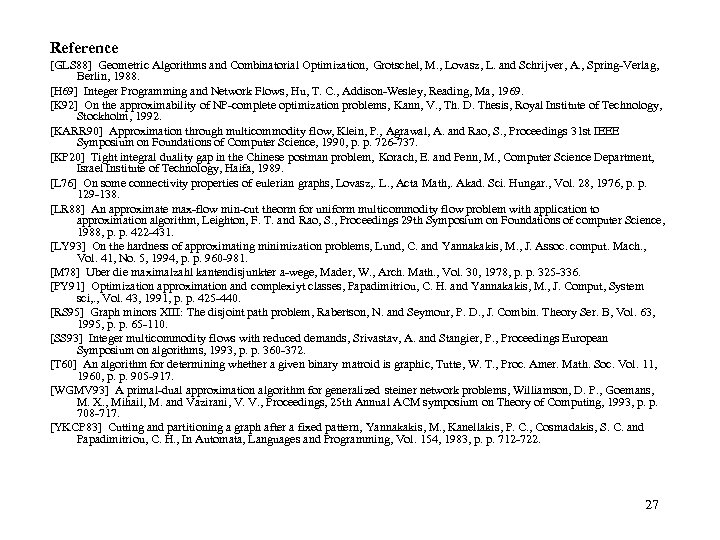Reference [GLS 88] Geometric Algorithms and Combinatorial Optimization, Grotschel, M. , Lovasz, L. and Schrijver, A. , Spring-Verlag, Berlin, 1988. [H 69] Integer Programming and Network Flows, Hu, T. C. , Addison-Wesley, Reading, Ma, 1969. [K 92] On the approximability of NP-complete optimization problems, Kann, V. , Th. D. Thesis, Royal Institute of Technology, Stockholm, 1992. [KARR 90] Approximation through multicommodity flow, Klein, P. , Agrawal, A. and Rao, S. , Proceedings 31 st IEEE Symposium on Foundations of Computer Science, 1990, p. p. 726 -737. [KP 20] Tight integral duality gap in the Chinese postman problem, Korach, E. and Penn, M. , Computer Science Department, Israel Institute of Technology, Haifa, 1989. [L 76] On some connectivity properties of eulerian graphs, Lovasz, . L. , Acta Math, . Akad. Sci. Hungar. , Vol. 28, 1976, p. p. 129 -138. [LR 88] An approximate max-flow min-cut theorm for uniform multicommodity flow problem with application to approximation algorithm, Leighton, F. T. and Rao, S. , Proceedings 29 th Symposium on Foundations of computer Science, 1988, p. p. 422 -431. [LY 93] On the hardness of approximating minimization problems, Lund, C. and Yannakakis, M. , J. Assoc. comput. Mach. , Vol. 41, No. 5, 1994, p. p. 960 -981. [M 78] Uber die maximalzahl kantendisjunkter a-wege, Mader, W. , Arch. Math. , Vol. 30, 1978, p. p. 325 -336. [PY 91] Optimization approximation and complexiyt classes, Papadimitriou, C. H. and Yannakakis, M. , J. Comput, System sci, . , Vol. 43, 1991, p. p. 425 -440. [RS 95] Graph minors XIII: The disjoint path problem, Rabertson, N. and Seymour, P. D. , J. Combin. Theory Ser. B, Vol. 63, 1995, p. p. 65 -110. [SS 93] Integer multicommodity flows with reduced demands, Srivastav, A. and Stangier, P. , Proceedings European Symposium on algorithms, 1993, p. p. 360 -372. [T 60] An algorithm for determining whether a given binary matroid is graphic, Tutte, W. T. , Proc. Amer. Math. Soc. Vol. 11, 1960, p. p. 905 -917. [WGMV 93] A primal-dual approximation algorithm for generalized steiner network problems, Williamson, D. P. , Goemans, M. X. , Mihail, M. and Vazirani, V. V. , Proceedings, 25 th Annual ACM symposium on Theory of Computing, 1993, p. p. 708 -717. [YKCP 83] Cutting and partitioning a graph after a fixed pattern, Yannakakis, M. , Kanellakis, P. C. , Cosmadakis, S. C. and Papadimitriou, C. H. , In Automata, Languages and Programming, Vol. 154, 1983, p. p. 712 -722. 27Thank you. 28